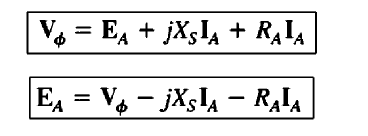# What is Synchronous Motor | Construction | Working Principle | Advantages |

### What is synchronous motor:

As the name suggests, the motor’s stator and rotor run at the synchronous speed, such a motor is called Synchronous motor. Typically, the speed of the stator (Rotating magnetic field) is exactly equal to the speed of the rotor. It runs at constant RPM irrespective of the load changes. Overexcited synchronous motors are mainly used for power factor correction in the transmission lines. However, in this article, we are going to study construction, Working principle, advantages, disadvantages and application of the synchronous motor.

Now you get a question is how the speed of the rotor is equal to the speed of the rotor?

Yes, the same will be achieved by providing the DC excitation to the rotor winding, here the synchronous motor receives two excitations, therefore it is called a doubly excited motor.

### Construction of Synchronous Motor:

Construction front, synchronous motor consists of the stator is a stationary part and rotor is a rotating part. The stator consists of a core and the slots to hold the armature winding similar to Synchronous generator.

The armature winding will be insulated by the varnish or paper. The stator’s core is made of silicon stamping with the laminated assembly which helps us to reduce the eddy current losses and hysteresis loss.

Rotor poles are projected type and they are mounted on the shaft of the motor. The rotor winding will be connected to a DC source with the help of slip rings.

Also, the speed of the motor is purely depending on the input supply frequency and poles.

### Working Principle:

For a simple understanding of the working principle of a synchronous motor, let we take a two-pole synchronous motor as shown in figure 1.1. The input rotor field current If creates a steady-state magnetic field Br and whose poles Nr and Sr. Now three-phase voltage will be applied to the stator winding and the same produce Rotating Magnetic field Bs and Whose poles are Ns and Ss.

i.e Nr is near to Ns. Now, pole Nr always tries to attract Ss and repulse Ns pole. In the worst case, the motor rotates a half-round by repulsing from Nr. After completion of half-round, the Nr reaches the SS hence Ss will be attracted by Nr and they will be locked magnetically. It means the rotor poles will tend to line up with the stator poles. Due to this reason, the synchronous motors are not the self-starting motor.

Therefore, the synchronous motor will start with the external machine, the machine helps us to reach the rotor to synchronous speed of the stator. After reaching the synchronous speed the external machine will be cut off and the synchronous motor will run constantly.

But in order to achieve continuous magnetic locking, the motor’s excitation needs to be given continuously.

The synchronous speed will be calculated by the below formula.

Ns=120f/P

Ns => synchronous speed

F => supply frequency

P = number of Poles.

### The Equivalent Circuit:

The equivalent circuit of a synchronous motor is exactly the same as a synchronous generator. Only one difference is the direction of armature current Ia is reversed. Because of the motoring operation, the synchronous motor’s current will be fed from the source.

Fig 1.2 gives you an exact idea about the current flow of the synchronous motor.

As before, the input connection of the stator winding will be either Y- or d-connected. Because of the change in direction of lA., the Kirchhoff’s voltage law equation for the equivalent circuit changes too. Writing a Kirchhoff’s voltage law equation for the new equivalent circuit yields.This is exactly the same as the equation for a generator, except that the sign on the current term has been reversed.

1. The speed of the motor is constant irrespective of the load changes.
2. The overexcited synchronous motor [leading power factor] can be used as a reactive power generator, the same principle is used in the transmission and distribution.
3. The efficiency of the Synchronous motor is high.
4. They are mechanically stable irrespective of the air gap.
5. Can be operated at variable power factor.

1. They are not self-starting motors
2. High cost and cost vs Kw is higher than the three-phase IM. i.e Siemens make 2HP 3 Phase IM costs around Rs. 5,000 but the same capacity motor in SM will be 20,000 rupees, almost 4 times of the IM.
3. Field winding needs a DC external source.
4. Slip rings are connected the excitation circuit with the rotor circuit. It causes additional maintenance cost.
5. Hunting
6. If the load torque is higher than the motoring torque means, the motor comes to standstill position, again we need to restart the motor.
Learn More:   V Curve & Inverted V Curve of Synchronous Motor Why?

#### Applications:

1. The constant speed property is used in machine tools, motor-gen set, clocks, blowers etc
2. Power factor correction circuit

• Does motor works when the field coil is open?

The motor stop rotating. But if the motor has damper squirrel cage bars, then the motor act as an induction motor.

• Does Synchronous motor acts as a Synchronous Generator?

Yes, this is the main feature of the motor, here power can be reversed in either side. The mechanical power can be converted into electrical power; such a machine is called a synchronous generator.

• Where we can buy SM?

Crompton and Siemens are the few manufactures producing Synchronous Motor. But, Crompton is cheaper than Siemens